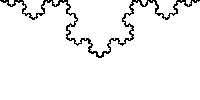## Friday, November 5, 2010

### Price Inflation - fractal

Inflation - a fractal explanation

Here I hypothesis price inflation is a fractal phenomena and is equivalent to the coastline paradox (the measurement of a coastline is related to the length of a the 'measuring stick') where the unit of 'money' is the coastline.

Update: 29th 11, 2012
If the fractal demonstrates the likes of price and quantity, supply and demand - as I have shown in my previous entries - then it should also be able to demonstrate inflation.
This entry has been a real challenge for me, but has been very existing : my thoughts maybe different, but they at least can't be over looked.

Just what is inflation?
Of course we have the textbook answer, a general increase in the average price level - over time, but to be fractal, it must be a universal definition, and go beyond prices of goods.
A fractal explanation will have to demonstrate the following - I am convinced that the general fractal does.
1. the increase in the descriptive value placed upon an 'unchanging' object
2. there must be devaluation of a kind of the measuring stick.
3. there must be a notion of equilibrium, where one gets used to the new nominal change or size

A fractal explanation for inflation
When we look at the  Koch Snowflake development/production (below), what is it that inflates - the area,, the number of 'triangles', or the perimeter - or nothing?Inflation and perimeter

I have become convinced that inflation is represented by the perimeter of the fractal, the reason being that the area of the Koch is finite, while the perimeter is infinite - as the fractal iterates (grows). Also note that area and perimeter are related through the base length of the triangle side too.

This came to me after thinking about what the perimeter length of an island, or the length of a piece of string. Fractal theory says both are infinite, and that the result depends on the actual length of the measuring stick. The shorter the stick, the larger the perimeter length: all while, at the same time the shape – the object – remains the same, or at equilibrium. This measuring problem is well described from around 2 minutes into the following: youtube clip: BBC, How long is a piece of string?

P= s.l
The perimeter (P) of the Koch snowflake fractal is calculated by multiplying number of sides (s) by length of a side (l).  The below diagram shows as the fractal iterates - and the shape develops towards equilibrium, at iteration 7 (plus or minus 2) - the perimeter of the snowflake rises exponentially to infinity. This is due to relationship between the diminishing length of each side, and the exponentially rising quantity of sides.This to me is also analogise to price inflation and the concept of value in economies: (Domestic) Production (GDP), where the measuring stick is units of currency or money.
Measuring an economies GDP should be the same principle as measuring an island, or the Koch snowflake's perimeter, only the measuring tool is money, or currency in this context.

To go any further we need to clear what money is? By definition, money should be - with the help of the circular flow of income model: Y=P=E - a liquid means of exchange, derived from income (Y), which comes from production (P), and will in turn be what exspended or spent (E).
It follows that if the Product (the production) of the Koch snowflake is the triangle bits; if so, then these bits represent 'money' as a means of exchange .
As the fractal grows (as domonstrated in the fractal zoom below) the sides and bits get smaller and smaller, while the perimeter gets larger and larger.the Koch fractal zoom - growth
If we were to stop the zoom at any time, we see the same 'snowflake' ahead, just like shape as earlier in the zoom: that is to say, in our analogy, the money looks the same, only the the numbers have changed. We still see an equilibrium shape.

With that, I conclude that inflation is natural, and that growth, development and now inflation are inextricably linked through the mechanics of the fractal.

However, it begs the question, what about printed or fiat money? Does it distort the reality? Is there such a thing as unnatural inflation?
It is my thinking that fiat money is another fractal of its own. If we print (more) money - than what our resources call for - it follows that we will shorten measuring tool more than what is natural, distorting the reality with an even longer perimeter, or larger (misleading) GDP.

Also, for inflation to be fractal, inflation has to be a universal concept: I am interested to learn that hotels now come with 6, 7, and possibly 8 stars to rate their quality.  Have hotels really changed so much to deserve more numbers than the ‘5 star’. Of course they have, but maybe the system is open to some distortion - 5 should be the ultimate. As the 'stars' increase or inflate above the original ‘5 star’, that 5 star loses value.  Other examples exist:  academic grade inflation - where the school leaving qualification creeps up and up, from a Degree to a Masters to where you need a PhD to work behind a bar.

We know, from experience, and from neo-classical economic's, that price inflation fits the above 'rules': (nominal) price's rise for the same object;  consumers get used to the new price's - there is new equilibrium; and finally there is a devaluation of money itself, when relating to its past value.

I also consider changes in models of car (for example) without any inovation or evolution, just the colour of style, as inflation - design inflation. The new car devalues the old just because it is has something different (no practicle) - even if they are basically the same car.

I am still of minds that there is more to this: there could be something in the quantity of 'triangles' to explore.

﻿